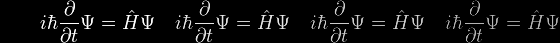# Iteration to Self-Consistency

It often happens that we need to solve an equation of the form a = f(a), where the precise form of the function f( ) depends on the problem in hand. Eboracus may well remember one such instance from his days as Chief Programmer at a UK financial institution, where an interest rate needed to be calculated subject to constraints that themselves depended on that very interest rate.

In these cases

• [Eqn 14e] would be recast as: a = ℓ / sinh(d/a)
• [Eqn 15] would be recast as: a = h / [cosh(d/a) – 1]

To solve such circular problems, we adopt a circular procedure, by guessing a plausible value of a, a0 say, and evaluating f(a0). These two will not be equal (unless we are incredibly lucky), so we calculate their average (arithmetic, geometric or harmonic, according to taste) a1 = [a0 +f(a0)]/2, say. We repeat the procedure for a1, a2, a3, ... , an until we observe that an and f(an) are equal to within the required number of significant figures. an is said to be self-consistent.

When this procedure works, it's said to be convergent. When it doesn't, it's said to be divergent, or maybe oscillatory. Successful convergence all too often depends on how good the initial guess was.